# Python W模式

``````with open(r'G:\PythonProject\学习\学习文件\date\c.txt', mode='wt', encoding='utf-8') as f:
pass``````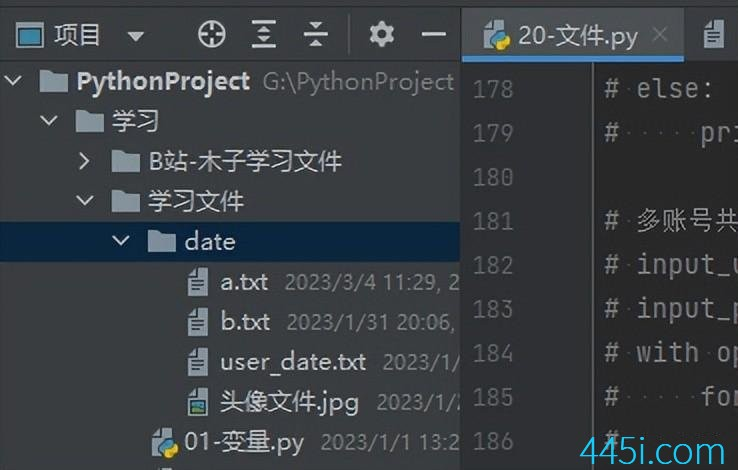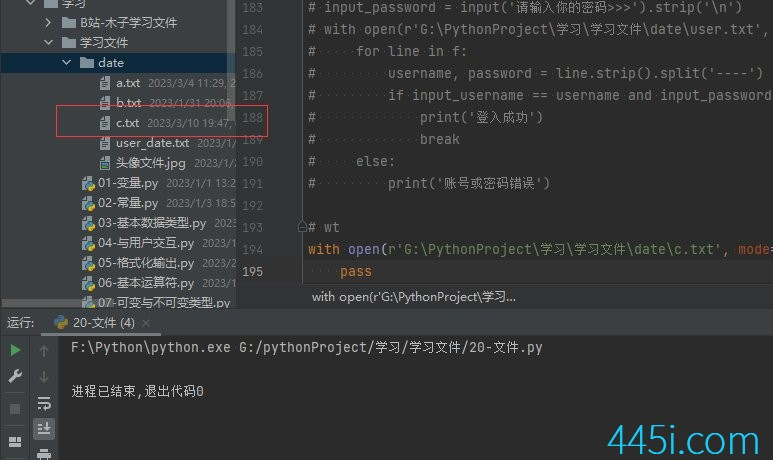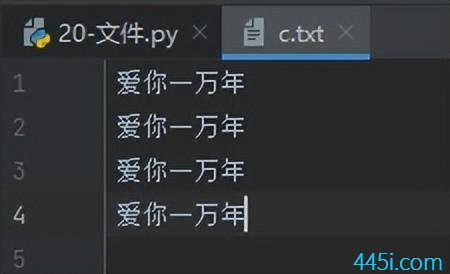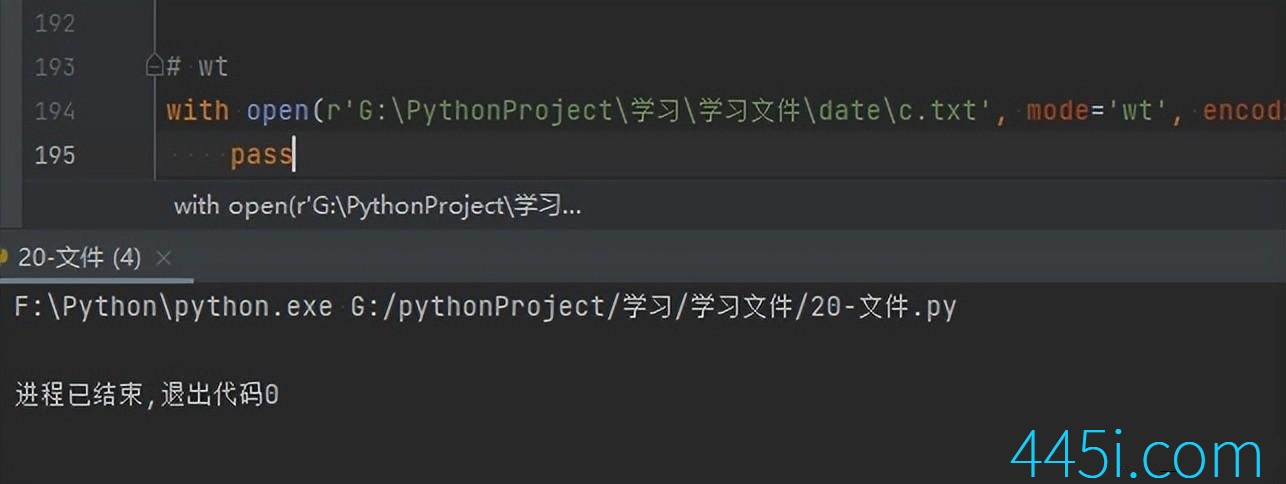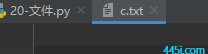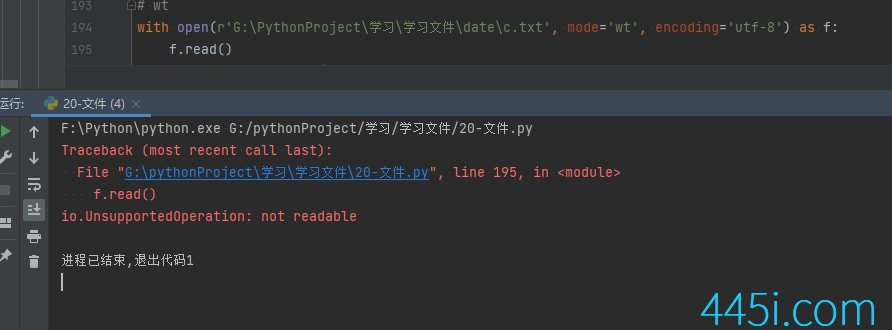``````with open(r'G:\PythonProject\学习\学习文件\date\c.txt', mode='wt', encoding='utf-8') as f:
f.write('晓看天色暮看云')``````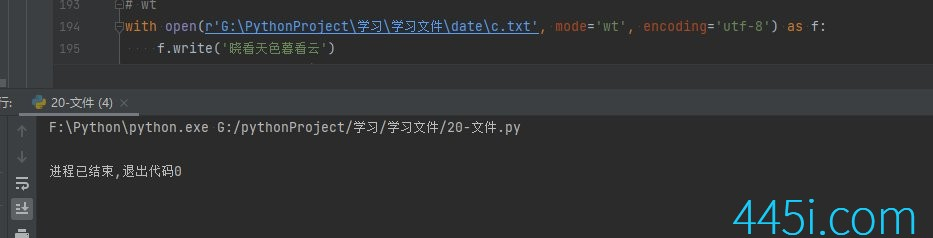``````with open(r'G:\PythonProject\学习\学习文件\date\c.txt', mode='wt', encoding='utf-8') as f:
f.write('晓看天色暮看云\n')``````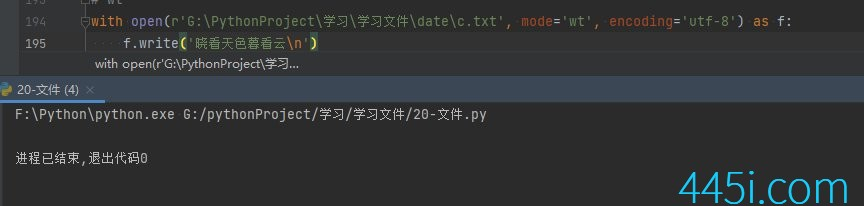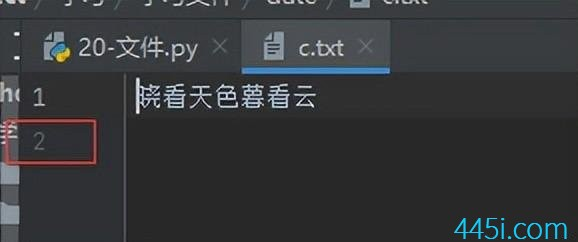``````with open(r'G:\PythonProject\学习\学习文件\date\c.txt', mode='wt', encoding='utf-8') as f:
f.write('晓看天色暮看云\n')
f.write('唧唧复唧唧\n')``````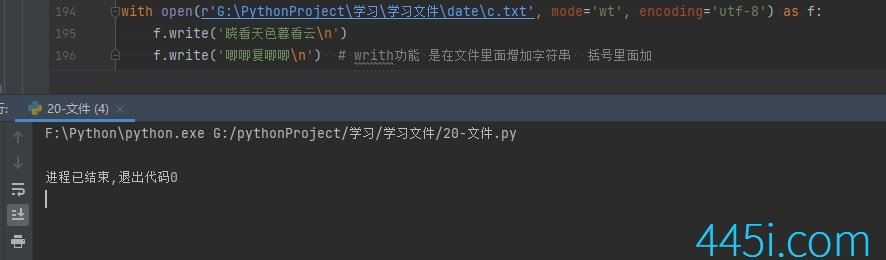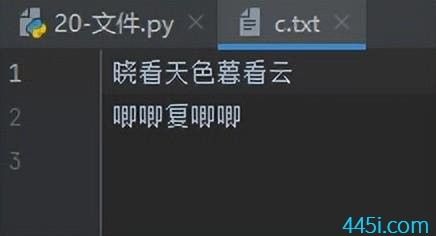### 觉得文章有用就打赏一下文章作者

#### 支付宝扫一扫打赏#### 微信扫一扫打赏